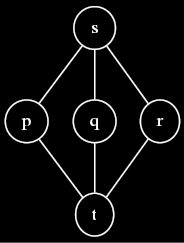# Lattice: Exercises

Try these exercises to test your knowledge of lattices.

## Distributivity

Does the lattice meet operator distribute over joins? In other words, for all lattices $$L$$ and all $$p, q, r \in L$$, is it necessarily true that $$p \wedge (q \vee r) = (p \wedge q) \vee (p \wedge r)$$? Prove your answer.

The following counterexample shows that lattice meets do not necessarily distribute over joins.<div><div>

comment: dot source:

digraph G { node = 0.1, height = 0.1 edge = “none”

rankdir = BT; t → p t → q t → r p → s q → s r → s } <div>%%

In the above lattice, $$p \wedge (q \vee r) = p \neq t = (p \wedge q) \vee (p \wedge r)$$. %%

## Common elements

Let $$L$$ be a lattice, and let $$J$$ and $$K$$ be two finite subsets of $$L$$ with a non-empty intersection. Prove that $$\bigwedge J \leq \bigvee K$$.

If $$J$$ and $$K$$ have a non-empty intersection, then there exists some lattice element $$p$$ such that $$p \in J$$ and $$p \in K$$. Since $$\bigwedge J$$ is a lower bound of $$J$$, we have $$\bigwedge J \leq p$$. Since $$\bigvee K$$ is an upper bound of $$K$$, we have $$p \leq \bigvee K$$. By transitivity, we have $$\bigwedge J \leq p \leq \bigvee K$$.

## Another inequality

Let $$L$$ be a lattice, and let $$J$$ and $$K$$ be two finite subsets of $$L$$ such that for all $$j \in J$$ and $$k \in K$$, $$j \leq k$$. Prove that $$\bigvee J \leq \bigwedge K$$.

Rephrasing the problem statement, we have that every element of $$J$$ is a lower bound of $$K$$ and that every element of $$K$$ is an upper bound of $$J$$. It the follows that for $$j \in J$$, $$j \leq \bigwedge K$$. Hence, $$\bigwedge K$$ is an upper bound of $$J$$, and therefore it is greater than or equal to the least upper bound of $$J$$: $$\bigvee J \leq \bigwedge K$$.

## The minimax theorem

Let $$L$$ be a lattice and $$A$$ an $$m \times n$$ matrix of elements of $$L$$. Prove the following inequality: $$\bigvee_{i=1}^m \bigwedge_{j=1}^n A_{ij} \leq \bigwedge_{j=1}^n \bigvee_{i=1}^m A_{ij}$$\$.

To get an intuitive feel for this theorem, it helps to first consider a small concrete instantiation. Consider the $$3 \times 3$$ matrix depicted below, with elements $$a,b,c,d,e,f,g,h$$, and $$i$$. The inequality instantiates to $$(a \wedge b \wedge c) \vee (d \wedge e \wedge f) \vee (g \wedge h \wedge i) \leq (a \vee d \vee g) \wedge (b \vee e \vee h) \wedge (c \vee f \vee i)$$. Why would this inequality hold?

$$\left[ \begin{array}{ccc} a & b & c \\ d & e & f \\ g & h & i \\ \end{array} \right]$$

Notice that each parenthesized expression on the left hand side of the inequality shares an element with each parenthesized expression on the right hand side of the inequality.This is true because the parenthesized expressions on the left hand side correspond to rows and the parenthesized expressions on the right hand side correspond to columns; each row of a matrix shares an element with each of its columns. The theorem proven in the Common elements exercise above then tells us that each parenthesized expression on the left hand side is less than or equal to each parenthesized expression on the right hand side.

Let $$J = \{ a \wedge b \wedge c, d \wedge e \wedge f, g \wedge h \wedge i \}$$ and $$K = \{ a \vee d \vee g, b \vee e \vee h, c \vee f \vee i \}$$. Then the hypothesis for the theorem proven in the Another inequality exercise holds, giving us $$\bigvee J \leq \bigwedge K$$, which is exactly what we wanted to prove. Extending this approach to the general case is straightforward. <div><div>

Parents: